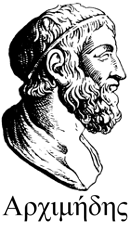MAIN NEWS STAFF SCIENCE STUDYDEPARTMENT OF EQUATION OF MATHEMATICAL PHYSICS SUSU RUSSIAN VERSION

# SCIENCE

### Conferences     |     Seminar

 The theory of partial differential equations are notions of parabolic, hyperbolic and elliptic equations, which are typical of the heat equation, wave equation and Laplace and Poisson equations. However, early in the last century, began to arise in the applications of partial differential equations, which can’t be classified. In particular, the eminent French mathematician H. Poincare (1854-1912) was opened on the system of equations that are solved with respect to time derivatives. Further equations and systems of equations that are solved for the selected derivative, appeared in the work of many mathematicians and mechanics (eg, such is the Navier – Stokes equations, modeling the dynamics of a viscous incompressible fluid, as a typical representative of which can take ordinary tap water.) The systematic study of these equations, which was initiated by the fundamental works of the outstanding Russian mathematician S. L. Sobolev (1908-1989), began in the middle of the last century. Then there was a tradition to name equations and systems of partial differential equations that are not allowed on the allocation of derivatives, equations of Sobolev type. Now these equations constitute a vast area of non-classical equations of mathematical physics, and the study of these equations are experiencing rapid flowering time – only books, all or part of them on, there are more than two dozen, not to mention the thousands of original articles. Initially, the study of equations that are unsolved for the selected derivative, was conducted mostly students of Sobolev, such as R. A. Aleksandryan, A. G. Kostyuchenko and G. A. Eskin, T. I. Zelenyak and many others. Their results have initiated the work V. N. Vragov, A. I. Kozhanov and S. G. Pyatkov on non-classical equations of mathematical physics. Currently, studies of such equations are concentrated in a few mathematical schools, both in Russia and abroad. The largest Russian schools are Irkutsk school, headed by N. A. Sidorov, Y. E. Boyarintsev, V. F. Chistyakov, Yekaterinburg school, led by I. V. Melnikova, Novosibirsk school, the brightest representatives of which, along with A. I. Kozhanov, S. G. Pyatkov are G. V. Demidenko and S. V. Uspensky and Chelyabinsk school, headed by G. A. Sviridyuk and V. E. Fedorov. Overseas studies are being conducted in Bologna, under the direction of A. Favini, in Osaka under A. Yagi and Austin under R. E. Showalter. The collective of department conducts an active scientific research in the field of Sobolev type equations, the purpose of which is to create a general theory and the development of specific applications. In this direction have already been some successes – solve two major problems formulated by pioneers. First, it is an explanation of the non-existence of the Cauchy problem for a Sobolev-type equation for arbitrary initial data even from a dense set, and second, an explanation of the strong instability of the solutions of these equations. On the work of applied research should include the study of optimal control and inverse problems, and the development of numerical methods for solving singular systems of ordinary differential equations, which is a typical representative of the Leontief interbranch balance model. In 2007, the in the department has been opened a postgraduate study on a specialty 01.01.02 – differential equations. Currently one person studying in graduate school.

### Conferences     |     Seminar

 MAIN || NEWS || STAFF || SCIENCE || STUDY